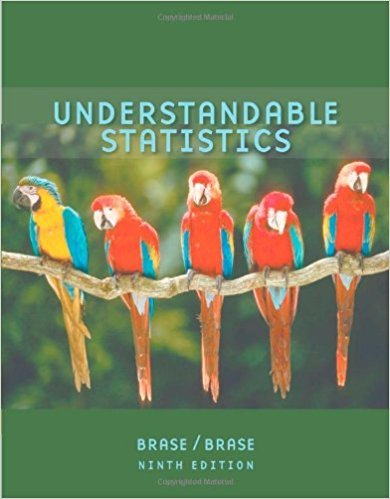×
×

# Solutions for Chapter 11.5: CHI-SQUARE AND F DISTRIBUTIONS## Full solutions for Understandable Statistics | 9th Edition

ISBN: 9780618949922Solutions for Chapter 11.5: CHI-SQUARE AND F DISTRIBUTIONS

Solutions for Chapter 11.5
4 5 0 349 Reviews
29
0
##### ISBN: 9780618949922

Chapter 11.5: CHI-SQUARE AND F DISTRIBUTIONS includes 9 full step-by-step solutions. This textbook survival guide was created for the textbook: Understandable Statistics, edition: 9. Since 9 problems in chapter 11.5: CHI-SQUARE AND F DISTRIBUTIONS have been answered, more than 35660 students have viewed full step-by-step solutions from this chapter. This expansive textbook survival guide covers the following chapters and their solutions. Understandable Statistics was written by and is associated to the ISBN: 9780618949922.

Key Statistics Terms and definitions covered in this textbook
• All possible (subsets) regressions

A method of variable selection in regression that examines all possible subsets of the candidate regressor variables. Eficient computer algorithms have been developed for implementing all possible regressions

• Backward elimination

A method of variable selection in regression that begins with all of the candidate regressor variables in the model and eliminates the insigniicant regressors one at a time until only signiicant regressors remain

• Bayes’ estimator

An estimator for a parameter obtained from a Bayesian method that uses a prior distribution for the parameter along with the conditional distribution of the data given the parameter to obtain the posterior distribution of the parameter. The estimator is obtained from the posterior distribution.

• Bivariate normal distribution

The joint distribution of two normal random variables

• Comparative experiment

An experiment in which the treatments (experimental conditions) that are to be studied are included in the experiment. The data from the experiment are used to evaluate the treatments.

• Components of variance

The individual components of the total variance that are attributable to speciic sources. This usually refers to the individual variance components arising from a random or mixed model analysis of variance.

• Confounding

When a factorial experiment is run in blocks and the blocks are too small to contain a complete replicate of the experiment, one can run a fraction of the replicate in each block, but this results in losing information on some effects. These effects are linked with or confounded with the blocks. In general, when two factors are varied such that their individual effects cannot be determined separately, their effects are said to be confounded.

• Consistent estimator

An estimator that converges in probability to the true value of the estimated parameter as the sample size increases.

• Convolution

A method to derive the probability density function of the sum of two independent random variables from an integral (or sum) of probability density (or mass) functions.

• Covariance matrix

A square matrix that contains the variances and covariances among a set of random variables, say, X1 , X X 2 k , , … . The main diagonal elements of the matrix are the variances of the random variables and the off-diagonal elements are the covariances between Xi and Xj . Also called the variance-covariance matrix. When the random variables are standardized to have unit variances, the covariance matrix becomes the correlation matrix.

• Defect concentration diagram

A quality tool that graphically shows the location of defects on a part or in a process.

• Density function

Another name for a probability density function

• Dispersion

The amount of variability exhibited by data

• Erlang random variable

A continuous random variable that is the sum of a ixed number of independent, exponential random variables.

• Event

A subset of a sample space.

• F distribution.

The distribution of the random variable deined as the ratio of two independent chi-square random variables, each divided by its number of degrees of freedom.

• Factorial experiment

A type of experimental design in which every level of one factor is tested in combination with every level of another factor. In general, in a factorial experiment, all possible combinations of factor levels are tested.

• Generator

Effects in a fractional factorial experiment that are used to construct the experimental tests used in the experiment. The generators also deine the aliases.

• Geometric mean.

The geometric mean of a set of n positive data values is the nth root of the product of the data values; that is, g x i n i n = ( ) = / w 1 1 .

• Goodness of fit

In general, the agreement of a set of observed values and a set of theoretical values that depend on some hypothesis. The term is often used in itting a theoretical distribution to a set of observations.

×